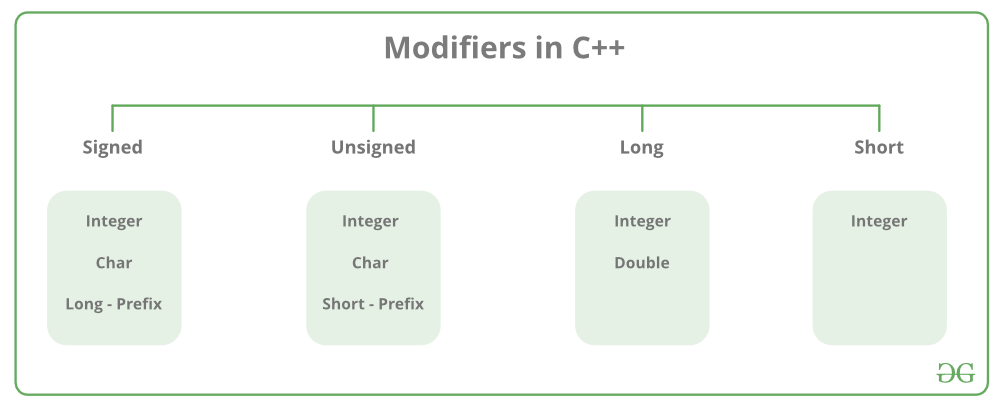# Difference between long int and long long int in C/C++

• Difficulty Level : Easy
• Last Updated : 13 Jun, 2022

All variables use data type during declarations to restrict the type of data to be stored. Therefore, we can say that data types are used to tell the variables the type of data it can store. Whenever a variable is defined in C++, the compiler allocates some memory for that variable based on the data type with which it is declared. Different data types require a different amount of memory.

Integer: The keyword used for integer data types is int. Integers typically require 4 bytes of memory space and range from -2147483648 to 2147483647.

Datatype Modifiers: As the name implies, datatype modifiers are used with the built-in data types to modify the length of data that a particular data type can hold.Below is a list of ranges along with the memory requirements and format specifiers on the 32-bit GCC compiler.

Long long takes the double memory as compared to long. But it can also be different on various systems. Its range depends on the type of application. The guaranteed minimum usable bit sizes for different data types:

• char: 8
• short:16
• int: 16
• long: 32
• long long: 64

The decreasing order is: long long >=long>=int>=short>=char

Program 1:

In various competitive coding platforms, the constraints are between 107 to 1018. Below is the program to understand the concept:

## C++

 `// C++ program to implement` `// the above approach` `#include ` `using` `namespace` `std;`   `// Driver code` `int` `main()` `{` `    ``// Value of p 10^5` `    ``int` `p = 100000;`   `    ``// Value of q 10^5` `    ``int` `q = 100000;`   `    ``int` `result = p * q;` `    ``cout << result << endl;` `    ``return` `0;` `}`

Output:

`1410065408`

As above, the output is not correct as int can’t store a 1010 value(out of its range). In this case, long long should be used.

Program 2:

Below is the C++ program to demonstrate how converting int to long long affects the output:

## C++

 `// C++ program to implement` `// the above approach` `#include ` `using` `namespace` `std;`   `// Driver code` `int` `main()` `{` `    ``// Value of p 10^5` `    ``int` `p = 100000;`   `    ``// Value of q 10^5` `    ``int` `q = 100000;`   `    ``long` `long` `int` `result = p * q;` `    ``cout << result << endl;` `    ``return` `0;` `}`

Output:

`1410065408`

Explanation: The above program gives the same output even after converting int to long long int because at first, the result is declared as long long. But before assigning the value of multiplication of p and q, it is already overflowed. Now, to prevent the overflow condition, it is required to convert the int result to the long long int before assigning the result value so that the overflow condition does not occur.

Program 3:

Below is the C++ program to implement the above approach:

## C++

 `// C++ program to implement` `// the above approach` `#include ` `using` `namespace` `std;`   `// Driver code` `int` `main()` `{` `    ``// Value of p 10^5` `    ``int` `p = 100000;`   `    ``// Value of q 10^5` `    ``int` `q = 100000;`   `    ``long` `long` `int` `result = (``long` `long` `int``)p * q;` `    ``cout << result << endl;` `    ``return` `0;` `}`

Output:

`10000000000`

Explanation: After this, it gives the correct output, which is 1010, which can be easily stored into a long long data type as the range is up to 1018.

My Personal Notes arrow_drop_up
Recommended Articles
Page :